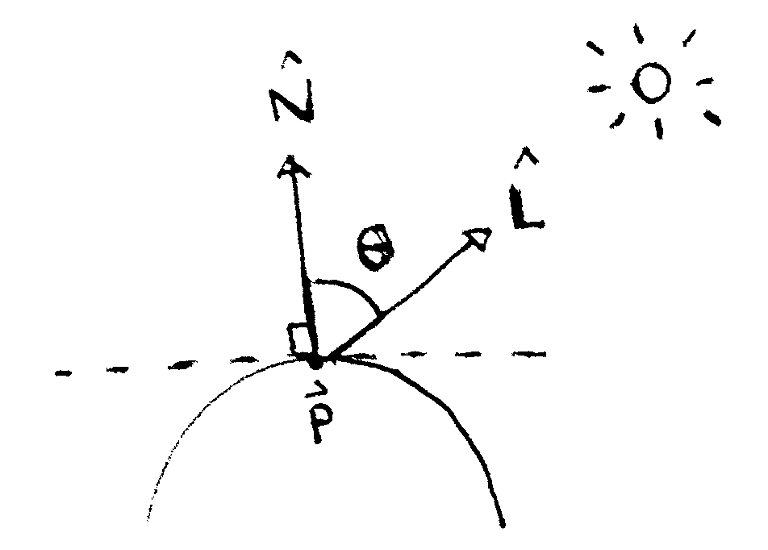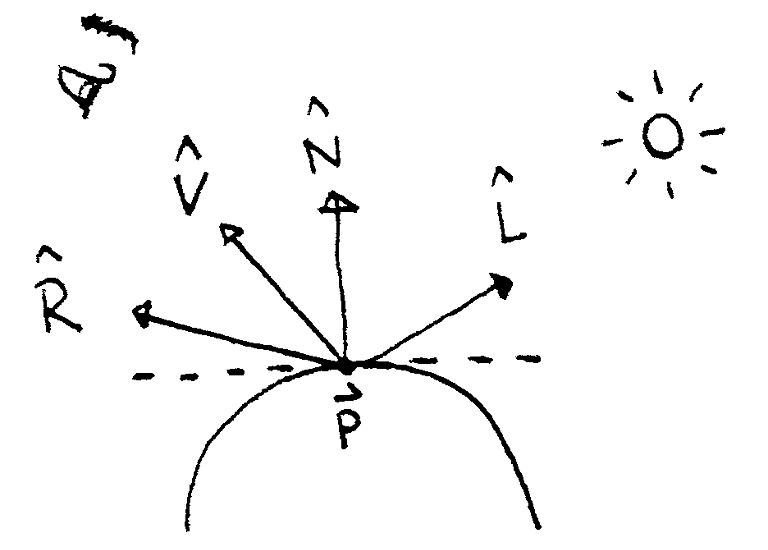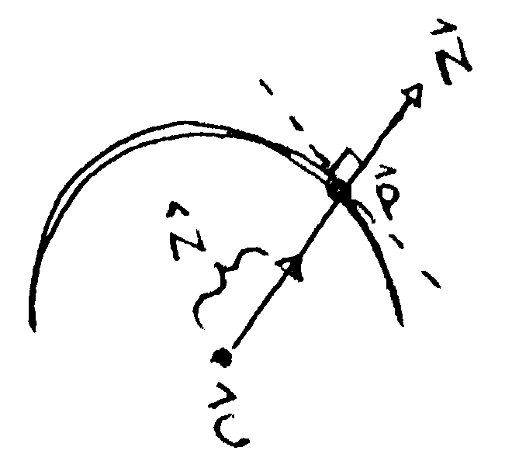# Build your own 3D renderer - Simulating illumination using Phong shading

We now know when the camera sees an object, but how do we know what color the corresponding pixel is? To answer this, we need to simulate how light interacts with the object. In general, this is a hard problem, but we can approximate it with local illumination. We will consider only the object and the lights in the scene, ignoring the effects of other objects in the scene. One way to do this is with the Phong reflectance model, which consists of three parts:

• The scene contains some ambient light, i_a (the i stands for light intensity). This approximates the light coming from other objects using a constant term. The object reflects some portion of this light, represented by a proportion, k_a, resulting in a contribution of k_a i_a.

• For each light in the scene, the object reflects some portion k_d of the light's intensity i_d. This is the diffuse component. A diffuse object scatters light in all directions.

The amount reflected depends on how the light hits the object: if the light hits the object directly, more is reflected than if the light grazes the object. So the contribution depends on the angle between the light vector hat bbL and the surface normal to the object at that point, hat bbN. The surface normal is the unit vector pointing directly away from the surface at that point.The diffuse component at a point vec bbp depends on the angle between two vectors. The first vector is the surface normal at the point, hat bbN. The second is the normalized vector between the point and the light, hat bbL.

The amount reflected is maximized when hat bbL and hat bbN point in the same direction, making this a perfect situation to use the dot product. Taking into acount that the object reflects a proportion k_d of the diffuse light, the diffuse component is then k_d i_d (hat bbN * hat bbL).

• For each light in the scene, there may also be a specular highlight that depends on the viewing angle. This highlight occurs because a reflective surface reflects light in a particular direction hat bbR. The more aligned the vector to the camera, hat bbV, is with the reflectance direction, the brighter that point appears to the viewer. The dot product comes in handy again.The specular highlight at a point vec bbp depends on the angle between the reflectance vector hat bbR and the viewing angle hat bbV. The reflectance vector depends on the light vector hat bbL.

The light intensity's specular component, i_s, is reflected in the direction of hat bbR, and only a proportion, k_s, is reflected. Finally, we include a shininess constant, alpha, making the specular highlight k_s i_s (hat bbV * hat bbR)^alpha. The larger alpha is, the shinier the material, and the smaller and brighter the highlight.

To compute the reflectance vector hat bbR, we can construct a parallelogram with the normal vector in the middle. As shown in the diagram below, hat bbR = 2(hat bbN * hat bbL) hat bbN - hat bbL.The light vector hat bbL is reflected across the normal direction, resulting in the reflectance vector hat bbR. Here, a parallelogram is formed by vec bbL and vec bbR, showing how the latter is calculated in terms of the former.

Adding all of these contributions from all lights, we get the color of the object at a given point, when viewed from a particular direction.

The last piece of the puzzle is how to calculate the surface normal for an object. For a sphere, the normal at a point vec bbp can be found by normalizing the vector between the center of the sphere, vec bbc, and the point: hat bbN = {vec bbp - vec bbc} / ||vec bbp - vec bbc||To find the surface normal hat bbN at point vec bbp, take the vector between vec bbp and the sphere's center vec bbc, then normalize it.